# SSC Reasoning Ability Practice Questions (Day-52)

Dear Aspirants, The SSC Exams are going on at national level. Millions of candidates have eagerly prepared for the ongoing SSC exams such as CGL, CHSL, MTS, CPO, JE etc. SSC exams are usually conducted by the Staff Selection Commission to recruit the eligible candidates for various Central government departments. Mostly the selection process for all the SSC exams consists of the single or double phase of written examination based on the examination. Due to that, the written exam consisted of a certain syllabus and exam pattern. By following those regulations, the written exam has been conducted through an online mode. Based on the latest updated exam pattern and syllabus, here we have drafted the Reasoning Ability questions on a daily basis. So aspirants start your practice session with us and mould your skill in a perfect shape.

Start Quiz

Direction (1): In the following question, select the number which can be placed at the sign of question mark (?) from the given alternatives.

1)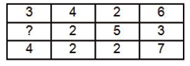(a) 3

(b) 4

(c) 5

(d) 6

Direction (2-3): How many triangles are there in the given figure?

2)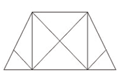(a) 14

(b) 16

(c) 18

(d) 20

3)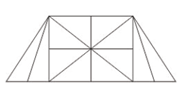(a) 18

(b) 24

(c) 26

(d) 28

Direction (4-5): In each of the following question below are given some statements followed by some conclusions. Taking the given statements to be true even if they seem to be at variance from commonly known facts, read all the conclusions and then decide which of the given conclusion logically follows the given statements.

4) Statements:

I) All cups are glasses.

II) Some cups are pens.

Conclusions:

I) Some pens are cups.

II) Some pens are glasses.

III) Some pens are not cups.

(a) Only conclusion (I) and (III) follow

(b) Only conclusion (II) and (III) follow

(c) Only conclusion (I) and (II) follow

(d) All conclusions follow

5) Statements:

I) No cities are countries.

II) No countries are villages.

Conclusions:

I) Some countries are city.

II) No villages are city.

(a) Only conclusion (I) follows

(b) Only conclusion (II) follows

(c) Both conclusion follow

(d) Neither conclusion (I) nor conclusion (II) follows

6) From the given options, which answer figure can be formed by folding the figure given in the question?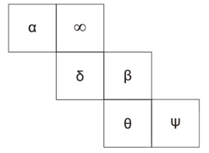(a)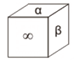(b)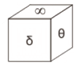(c)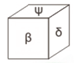(d)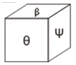7) From the given options, which answer figure can be formed by folding the figure given in the question?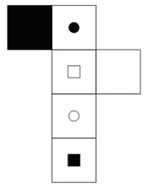(a)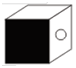(b)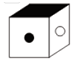(c)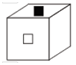(d)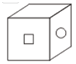8) In the given figure, how many papers are also hard?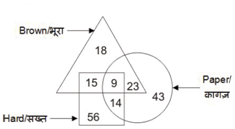(a) 14

(b) 23

(c) 37

(d) 32

9) In the given figure, how many keys are duplicate but not steel?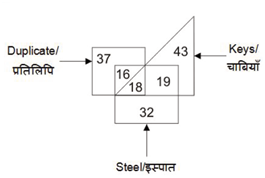(a) 16

(b) 18

(c) 0

(d) 34

10) In the given figure, how many leather bags are not red?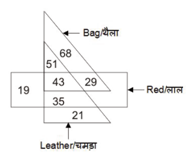(a) 29

(b) 76

(c) 43

(d) 51

3 + 4 + 2 + 6 = 15

4 + 2 + 2 + 7 = 15

5 + 2 + 5 + 3 = 15

16 triangles

26 triangles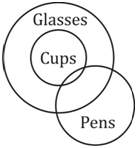I) √

II) √

III)  ×

Only conclusion I and II follow.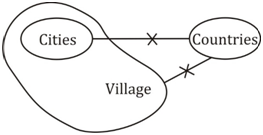I) ×

II) ×

Neither conclusion I nor conclusion II follows.

Opposite faces are

Β → ∝

∞ → θ

δ → Ψ

Option (d) can be formed.

Opposite faces are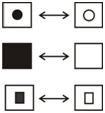Option (d) can be formed.

Papers which are hard = 9 + 14

= 23

O keys are duplicate but not steel.